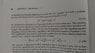# Damped Oscillators and Binomial theorem step

• I
• Teachme

#### Teachme

I uploaded a picture of what I am stuck on. I understand the equation of motion 3.4.5a for a damped oscillator but I don't understand how to use binomial theorem to get the expanded equation 3.4.5b. I am no where near clever enough to figure this one out. I know how to use binomial theorem to expand something with two terms but the fact that this has three terms and is only to the first power throws me off and I don't know how to apply the binomial theorem. If anyone could give me any hints, I would very much appreciate it.

#### Attachments

•IMG_20160415_131247610.jpg
39.9 KB · Views: 424
I uploaded a picture of what I am stuck on. I understand the equation of motion 3.4.5a for a damped oscillator but I don't understand how to use binomial theorem to get the expanded equation 3.4.5b. I am no where near clever enough to figure this one out. I know how to use binomial theorem to expand something with two terms but the fact that this has three terms and is only to the first power throws me off and I don't know how to apply the binomial theorem. If anyone could give me any hints, I would very much appreciate it.

what i can gather that you are writing the equivalent expressions for a 2nd order operator equation composed of two first order multiples and if you multiply they come out to be same...
the author refers this operation to a binomial expansion - may be the 'mathematicians' may be calling this by the name ' binomial.'
though its factorizing the quadratic equation in D. D being a differential operator-being a physics person we usually use maths as a tool.
but you can see Abramowitch and Stegun - mathematical functions and there is good info on binomials.

the two roots are say D1 and D2 and the two bracketed terms identically goes to zero.

I uploaded a picture of what I am stuck on. I understand the equation of motion 3.4.5a for a damped oscillator but I don't understand how to use binomial theorem to get the expanded equation 3.4.5b. I am no where near clever enough to figure this one out. I know how to use binomial theorem to expand something with two terms but the fact that this has three terms and is only to the first power throws me off and I don't know how to apply the binomial theorem. If anyone could give me any hints, I would very much appreciate it.

The factoring has nothing to do with the binomial theorem. All that it is used is: $(a^2-b^2)=(a+b)(a-b)$.

The factoring has nothing to do with the binomial theorem. All that it is used is: $(a^2-b^2)=(a+b)(a-b)$.
Hey Mathman,
thanks for your response. I think it is obvious that it is a2-b2=(a+b)(a-b) going from the second equation(3.4.5b) to the first equation(3.3.5a) but I don't see how it is ovbious going from equation(3.3.5a) to equation(3.4.5b).

Thanks for you help, much appreciated.

what i can gather that you are writing the equivalent expressions for a 2nd order operator equation composed of two first order multiples and if you multiply they come out to be same...
the author refers this operation to a binomial expansion - may be the 'mathematicians' may be calling this by the name ' binomial.'
though its factorizing the quadratic equation in D. D being a differential operator-being a physics person we usually use maths as a tool.
but you can see Abramowitch and Stegun - mathematical functions and there is good info on binomials.

the two roots are say D1 and D2 and the two bracketed terms identically goes to zero.

Thank you drvrm,
I will look into that book.

Thanks again for the help, I really appreciate it.

Hey Mathman,
thanks for your response. I think it is obvious that it is a2-b2=(a+b)(a-b) going from the second equation(3.4.5b) to the first equation(3.3.5a) but I don't see how it is ovbious going from equation(3.3.5a) to equation(3.4.5b).

Thanks for you help, much appreciated.
The author had something in mind.
$D^2+2\gamma D+\omega_0^2 = D^2+2\gamma D+\gamma^2+\omega_0^2-\gamma^2 = (D+\gamma )^2-(\gamma^2-\omega_0^2)$

The author had something in mind.
$D^2+2\gamma D+\omega_0^2 = D^2+2\gamma D+\gamma^2+\omega_0^2-\gamma^2 = (D+\gamma )^2-(\gamma^2-\omega_0^2)$
Oh wow, ok, I see now. You are too smart. Thanks soo much! I Wouldn't have figured that one out!